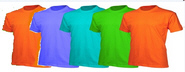Name:    1G3.2

Multiple Choice
Identify the choice that best completes the statement or answers the question.

1.

Which two shapes show the same equally colored parts of the pie charts?
 a.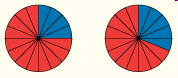c.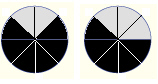b.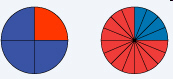2.

Which pictures shows half are different?
 a.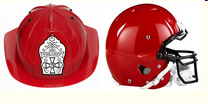b.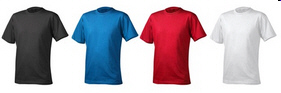3.

Which of the following pictures shows that two halves of the objects are the same?
 a.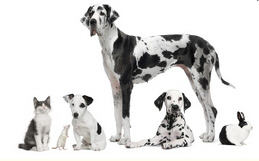b.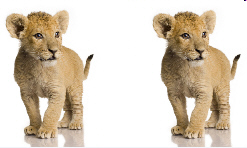4.

Which of the following shows one quarter of the items is different?
 a.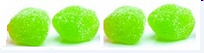b.5.

Which of the following pictures shows one half of a group?
 a.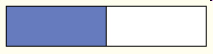b.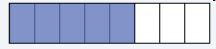6.

Which of the following shows one whole group of something?
 a.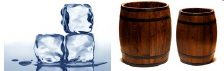b.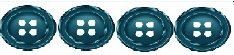7.

Which of the following shows two halves of the objects are brown?
 a.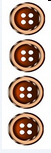b.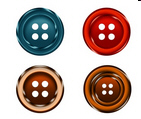8.

Which of the following pictures shows one half of the buttons are red?
 a.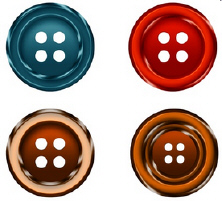b.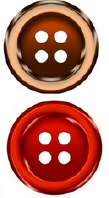9.

Which of the following shows that two quarters of the items are blue?
 a.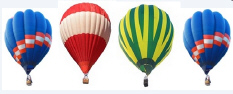b.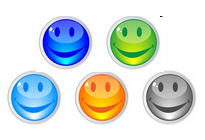10.

Which of the following objects show four fourths of the objects are the same?
 a.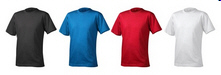c.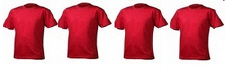b.